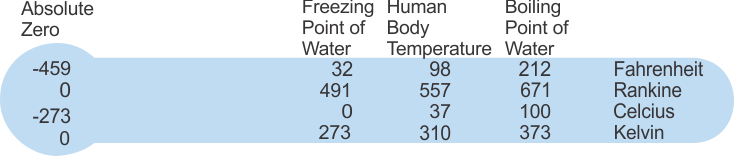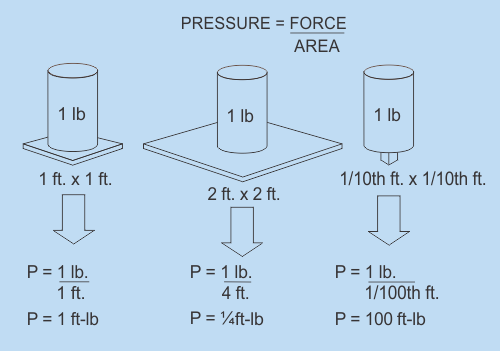### Definitions

#### Temperature

Imperial
Metric
Fahrenheit
Rankine
Celsius
Kelvin

Temperature scales are a way of describing how hot a substance is. A lump of matter contains energy. There are many forms of energy. One of them is Kinetic energy and measuring temperature is a way of measuring how furiously the molecules contained in a lump of substance are moving about. This molecular activity causes what we perceive as the temperature of an object. A refrigeration mechanic must be able to deal with temperatures in various scales. Traditionally the Imperial system has been used (Fahrenheit degrees) and a whole series of familiar capacity measurements like Horse Power, BTU's, Tons, and PSI have been the norm. However the Metric system, which is designed to be easier to work with has found wide spread use globally. In both systems there are standard and absolute temperature scales. Try experimenting with the above temperature converter. Type a value in any one of the input boxes and click on the Convert Button. Here are several interesting values to try: -40 °F, 0 °R, 40 °F, 373 °K, 21 °C

#### Celsius

In theory the Celsius scale should be much easier to work with. It is based on calling the freezing point of water zero and the boiling point of water 100. There are therefore 100 degrees between those 2 points. The Celsius temperature scale is also referred to as the "Centigrade" scale. Centigrade means "consisting of or divided into 100 degrees. I wonder what a comfortable room of 70° F would be in Celsius? If you don't happen to have a conversion calculator at your disposal you can always rely on the following 2 formulae:#### Kelvin

Scientists use the Kelvin scale, which is based on the Celsius scale, but has no negative numbers. Instead of basing it's zero point on the freezing point of water, it bases it's zero point on Absolute Zero. which is the theoretical temperature where all heat has been removed from a substance. Hence any amount of heat added creates a positive temperature. Negative numbers can mess up a scientist's mathematical calculations. You will find that in refrigeration, we too must use absolute temperature scales for some things. At Absolute Zero scientists believe that molecular motion would stop.

#### Rankine

Rankine is the Imperial version of an absolute temperature scale. Add 460 degrees to Fahrenheit temperatures to obtain the Rankine temperature. Input 0° in the Rankine box on the calculator above and you will see why.#### Heat

Temperature is a qualitative measurement. Heat is a quantitative measurement. The temperature "quality" of a object describes how hot it is but not the total amount of heat it actually contains. Here's a silly example which makes clear the distinction. Let's say we have two blocks of iron. One is a mere cubic inch [16 cubic cm] the other is 10 feet cubed. [30,000,000 cubic cm] We heat each of them to 150 °F [65 °C] and you verify this with some sort of thermometer. They both have the same temperature but do they both contain the same amount of heat? When you throw the little cube in your swimming pool nothing noticeable happens to the temperature of the pool water but when you toss in the huge iron chunk the pool water can be measured to rise somewhat over time. If there was a noticeable amount of heat transfer from the large chunk of iron but not from the small chunk of iron then surely the large chunk contained more heat than the small one even though they were at the same temperature. The temperature of an object is a reflection of the kinetic energy of the atoms or molecules that make it up. Fast molecules = high kinetic energy = high temperature. On the other hand heat represents the total amount of kinetic energy in an object. Heat is measured in BTU's.[or joules] Recall that 1 BTU is the amount of heat required to change the temperature of 1 Lb. [0.45 kilograms] of water through 1° F. [0.5 °C] So it would take 2 BTU to raise the temperature of 2 Lb. of water through 1 °F. And it would take 30 BTU to raise the temperature of 3 Lb. of water by 10 °F. BTU's (or their metric counterparts) Larger quantities of heat in the Imperial system are described with the term Ton. 12,000 BTU = 1 Ton. [3.52 kW] A building might have a 3 Ton [10.5 kW"] Air Conditioning system which would be equivalent to 36,000 BTUH. [10.5 kW]

#### Specific Heat

Specific heat capacity is the amount of heat required to change temperature of a given quantity of a substance by one degree. Specific heat may be measured in Btu/lb °F or kJ/kg °K. Different substances have different heat holding capabilities and thermal properties. Just because 1 Lb. of water will change precisely through 1° F when 1 BTU is applied to it does not necessarily mean that the same thing will happen with 1 Lb. of copper or 1 Lb. of steel or 1 Lb. of ice cream. There is a need to be able to specify those differences and the method utilized is to compare all substances to water. Water is given a specific heat value of 1. This means that 1 BTU is required to change the temperature of 1 Lb. of water through 1° F. The specific heat of water can also be described in the metric system. The metric specific heat of water is 1 calorie per gram per degree Celsius. This value also works out to 1. In other words it would take 1 calorie of heat to raise the temperature of 1 gram of water through 1 degree Celsius. Specific heat is a dimensionless quantity. It is purely a number having no unit of measurement associated with it. In Refrigeration specific heat values are used to calculate capacity requirements for refrigerating known quantities of product. For example one might need to be able to select refrigeration equipment capable of cooling 5000 Lb. [2268 kilograms] of beef from 55 °F [12.8 °C] to -20° F.[-29 °C] A calculation like that must take into consideration the fact that the specific heat of a substance usually is different above and below it's freezing point.

#### Latent Heat

Latent Heat is the heat given up or absorbed by a substance as it changes state. It is called latent because it is not associated with a change in temperature. Each substance has a characteristic latent heat of fusion, latent heat of vapourization, latent heat of condensation and latent heat of sublimation.#### Sensible Heat

Sensible Heat is associated with a temperature change, as opposed to latent heat. This is so-called because it can be sensed by humans. If the air in a building was to be heated from 60 °F [15.5 °C] to 70 °F [21 °C] only sensible heat would be involved. However, if the air in a building was to be cooled from 80 °F [26.7 °C] to 70 °F [21 °C] and humidity was to be removed from the air at the same time, then both sensible and latent heats would be involved.

#### Insulator

Electrical wires are coated with an insulating material so electricity stays in the conductor (wire). Thermal insulation on the other hand tries to keep heat from transferring. Thermal insulation does not stop heat transfer, it only slows down the rate of transfer. The greater the amount and quality of insulation, the greater the insulating effect and the slower is the thermal transfer. There is insulation inside cooler and freezer walls and in the perimeter walls of conditioned spaces. If fiberglass batting is being used it should be noted that the glass fibers are actually a poor insulator. It is the tiny pockets of trapped air in-between the fibers that actually are responsible for the insulating effect.

The chart below shows the specific heat values of several materials. Notice the very small specific heat value that copper has. This means it would take a mere .09 BTU [0.1 kJ] to raise 1 Lb. [0.45 kG] of copper through 1 °F. [0.5 °C] Copper has a bigger temperature change for the same heat input compared to many other materials. Copper transfers heat readily and would not make a very good insulator, it conducts heat too well. The smaller the specific heat number, the better of a conductor a material is. You can see why heat transfer devices like evaporators and condensers are made from materials like aluminum and copper.

Material Specific Heat (Btu/Lb. °F)
as well as (kcal/kg °C)
Specific Heat (calorie/gram °C)
as well as (kcal/kg °C)
Specific Heat (kJ/kg K)
Water 1 1 4.19
Ice less than 32 °F [0 °C] 0.49 0.49 2.09
Wood max 0.48 0.48 2.01
Ice     -4 °F [-20 °C] 0.47 0.47 1.97
Ice   -40 °F [-40 °C] 0.43 0.43 1.8
Ice -112 °F [-80 °C] 0.35 0.35 1.47
Wood min 0.32 0.32 1.34
Air 0.24 0.24 1.01
Concrete 0.23 0.2 0.8
Aluminum 0.22 0.22 0.9
Glass 0.20 0.2 0.84
Iron 0.12 0.11 0.45
Copper 0.09 0.09 0.39

Pressure is what occurs when a force is applied over an area. More specifically, pressure is the ratio of the force acting on a surface to the area of the surface. The equation for pressure represents this rather straightforwardly; P=F/A This equation means that Pressure equals Force divided by Area. Let's look at a couple of very simple examples. As is demonstrated in the sketches below, the same weight can exert completely different pressures depending on how much surface area it is spread out over. Note that in the Imperial System when you multiply FT by Lbs you get a unit called FT Lb. (pronounced Foot Pounds) This is a legitimate unit of pressure. However refrigeration pressures in the Imperial System are measured in pounds per square inch not pounds per square foot. This is abbreviated to PSI. Refrigeration gauges are zeroed to 1 Atm pressure and the units are then called PSIG. (as in PSI Gauge) The calculations shown in the metric picture yield pressure units in kg/m2 (kilograms per meter squared). This is also a legitimate unit of pressure however kPa (kilopascals) are the pressure units that you will see on Metric refrigeration gauges. As with temperature, pressure has many different scales that can be used and can be described with the Imperial system or the Metric system. We seldom deal with gravitational forces as shown in the diagram although it is an important concept to be aware of. Rather, we deal with the pressures and temperatures of gases and that is what the next section is all about.